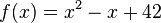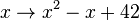# Anonymous function facts for kids

Kids Encyclopedia Facts

In computer science and mathematics, an anonymous function is a function with no name. Usually, a function is written like:$f(x) = x^2 - x + 42$. It can be written anonymously as$x \rightarrow x^2 - x + 42$. Anonymous functions exist in most functional programming languages and most other programming languages where functions are values.

## Examples in some programming languages

Each of these examples show a nameless function that multiplies two numbers.

### Python

Uncurried (not curried):

(lambda x, y: x * y)


Curried:

(lambda x: (lambda y: x * y))


When given two numbers:

>>>(lambda x: (lambda y: x * y)) (3) (4)
12


Curried:

\x -> \y -> x * y

\x y -> x * y


When given two numbers:

>>> (\x -> \y -> x * y) 3 4
12


The function can also be written in point-free (tacit) style:

(*)


### Standard ML

Uncurried:

fn (x, y) => x * y


Curried:

fn x => fn y => x * y


Point-free:

(op *)


### JavaScript

Uncurried:

(x, y) => x * y

function (x, y) {
return x * y;
}


Curried:

x => y => x * y

function (x) {
return function (y) {
return x * y;
};
}


### Scheme

Uncurried:

(lambda (x y) (* x y))


Curried:

(lambda (x) (lambda (y) (* x y)))


Point-free:

*

### C++ 11

Uncurried:

[](int x, int y)->int
{
return x * y;
};


Curried:

[](int x)
{
return [=](int y)->int
{
return x * y;
};
};


### C++ Boost

Uncurried:

_1 * _2


Note: What you need to write boost lambda is to include <boost/lambda/lambda.hpp> header file, and using namespace boost::lambda, i.e.

#include <boost/lambda/lambda.hpp>
using namespace boost::lambda;


## Related pagesAnonymous function Facts for Kids. Kiddle Encyclopedia.